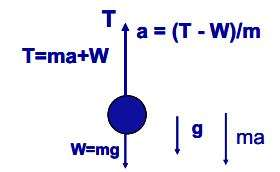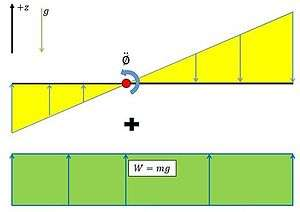# D'Alembert's principleJean d'Alembert (1717—1783)

D'Alembert's principle, also known as the Lagrange–d'Alembert principle, is a statement of the fundamental classical laws of motion. It is named after its discoverer, the French physicist and mathematician Jean le Rond d'Alembert. It is the dynamic analogue to the principle of virtual work for applied forces in a static system and in fact is more general than Hamilton's principle, avoiding restriction to holonomic systems. A holonomic constraint depends only on the coordinates and time. It does not depend on the velocities. If the negative terms in accelerations are recognized as inertial forces, the statement of d'Alembert's principle becomes The total virtual work of the impressed forces plus the inertial forces vanishes for reversible displacements. The principle does not apply for irreversible displacements, such as sliding friction, and more general specification of the irreversibility is required.

The principle states that the sum of the differences between the forces acting on a system of mass particles and the time derivatives of the momenta of the system itself along any virtual displacement consistent with the constraints of the system, is zero. Thus, in symbols d'Alembert's principle is written as following,whereis an integer used to indicate (via subscript) a variable corresponding to a particular particle in the system,is the total applied force (excluding constraint forces) on the-th particle,is the mass of the-th particle,is the acceleration of the-th particle,together as product represents the time derivative of the momentum of the-th particle, andis the virtual displacement of the-th particle, consistent with the constraints.

This above equation is often called d'Alembert's principle, but it was first written in this variational form by Joseph Louis Lagrange. D'Alembert's contribution was to demonstrate that in the totality of a dynamic system the forces of constraint vanish. That is to say that the generalized forcesneed not include constraint forces. It is equivalent to the somewhat more cumbersome Gauss's principle of least constraint.

## General case with changing masses

The general statement of d'Alembert's principle mentions "the time derivatives of the momenta of the system". The momentum of the i-th mass is the product of its mass and velocity:and its time derivative is.

In many applications, the masses are constant and this equation reduces to,

which appears in the formula given above. However, some applications involve changing masses (for example, chains being rolled up or being unrolled) and in those cases both termsandhave to remain present, giving## Derivation for special cases

To date, nobody has shown that D'Alembert's principle is equivalent to Newton's Second Law. This is true only for some very special cases e.g. rigid body constraints. However, an approximate solution to this problem does exist.

Consider Newton's law for a system of particles, i. The total force on each particle iswhereare the total forces acting on the system's particles,are the inertial forces that result from the total forces.

Moving the inertial forces to the left gives an expression that can be considered to represent quasi-static equilibrium, but which is really just a small algebraic manipulation of Newton's law:Considering the virtual work,, done by the total and inertial forces together through an arbitrary virtual displacement,, of the system leads to a zero identity, since the forces involved sum to zero for each particle.The original vector equation could be recovered by recognizing that the work expression must hold for arbitrary displacements. Separating the total forces into applied forces,, and constraint forces,, yieldsIf arbitrary virtual displacements are assumed to be in directions that are orthogonal to the constraint forces (which is not usually the case, so this derivation works only for special cases), the constraint forces do no work. Such displacements are said to be consistent with the constraints. This leads to the formulation of d'Alembert's principle, which states that the difference of applied forces and inertial forces for a dynamic system does no virtual work:.There is also a corresponding principle for static systems called the principle of virtual work for applied forces.

## D'Alembert's principle of inertial forces

D'Alembert showed that one can transform an accelerating rigid body into an equivalent static system by adding the so-called "inertial force" and "inertial torque" or moment. The inertial force must act through the center of mass and the inertial torque can act anywhere. The system can then be analyzed exactly as a static system subjected to this "inertial force and moment" and the external forces. The advantage is that, in the equivalent static system one can take moments about any point (not just the center of mass). This often leads to simpler calculations because any force (in turn) can be eliminated from the moment equations by choosing the appropriate point about which to apply the moment equation (sum of moments = zero). Even in the course of Fundamentals of Dynamics and Kinematics of machines, this principle helps in analyzing the forces that act on a link of a mechanism when it is in motion. In textbooks of engineering dynamics this is sometimes referred to as d'Alembert's principle.

### Example for 1D motion of a rigid bodyFree body diagram of a wire pulling on a mass with weight W, showing the d’Alembert inertia “force” ma.

To illustrate the concept of d'Alembert's principle, let's use a simple model with a weight, suspended from a wire. The weight is subjected to a gravitational force,, and a tension forcein the wire. The mass accelerates upward with an acceleration. Newton's Second Law becomesor. As an observer with feet planted firmly on the ground, we see that the forceaccelerates the weight,, but, if we are moving with the wire we don’t see the acceleration, we feel it. The tension in the wire seems to counteract an acceleration “force”or.Free body diagram depicting an inertia moment and an inertia force on a rigid body in free fall with an angular velocity.

### Example for plane 2D motion of a rigid body

For a planar rigid body, moving in the plane of the body (the xy plane), and subjected to forces and torques causing rotation only in this plane, the inertial force iswhereis the position vector of the centre of mass of the body, andis the mass of the body. The inertial torque (or moment) iswhereis the moment of inertia of the body. If, in addition to the external forces and torques acting on the body, the inertia force acting through the center of mass is added and the inertial torque is added (acting around the centre of mass is as good as anywhere) the system is equivalent to one in static equilibrium. Thus the equations of static equilibriumhold. The important thing is thatis the sum of torques (or moments, including the inertial moment and the moment of the inertial force) taken about any point. The direct application of Newton's laws requires that the angular acceleration equation be applied only about the center of mass.

### Dynamic equilibrium

D'Alembert's form of the principle of virtual work states that a system of rigid bodies is in dynamic equilibrium when the virtual work of the sum of the applied forces and the inertial forces is zero for any virtual displacement of the system. Thus, dynamic equilibrium of a system of n rigid bodies with m generalized coordinates requires that is to befor any set of virtual displacements δqj. This condition yields m equations,which can also be written asThe result is a set of m equations of motion that define the dynamics of the rigid body system.

## References

1. Lanczos, Cornelius (1970). The Variational Principles of Mechanics (4th ed.). New York: Dover Publications Inc. p. 92. ISBN 0-486-65067-7.
2. Cornelius Lanczos (1970). p. 90. ISBN 0-486-65067-7.
3. Udwadia, F. E.; Kalaba, R. E. (2002). "On the Foundations of Analytical Dynamics" (PDF). Intl. Journ. Nonlinear Mechanics. 37 (6): 1079–1090. Bibcode:2002IJNLM..37.1079U. doi:10.1016/S0020-7462(01)00033-6.
4. Arnold Sommerfeld (1956), Mechanics: Lectures on Theoretical Physics, Vol 1, p. 53
5. Rebhan, Eckhard (2006). "Exkurs 5.1: Ableitung des d'Alembert Prinzips". Mechanik. Theoretische Physik. Heidelberg, Germany: Spektrum Akademischer Verlag. ISBN 978-3-8274-1716-9.
6. Torby, Bruce (1984). "Energy Methods". Advanced Dynamics for Engineers. HRW Series in Mechanical Engineering. United States of America: CBS College Publishing. ISBN 0-03-063366-4.
7. Jong, Ing-Chang (2005). "Improving Mechanics of Materials". Teaching Students Work and Virtual Work Method in Statics:A Guiding Strategy with Illustrative Examples (PDF). 2005 American Society for Engineering Education Annual Conference & Exposition. Retrieved June 24, 2014.
8. Weisshaar, Terry (2009). Aerospace Structures - an Introduction to Fundamental Problems. Purdue University. pp. 50, 58.